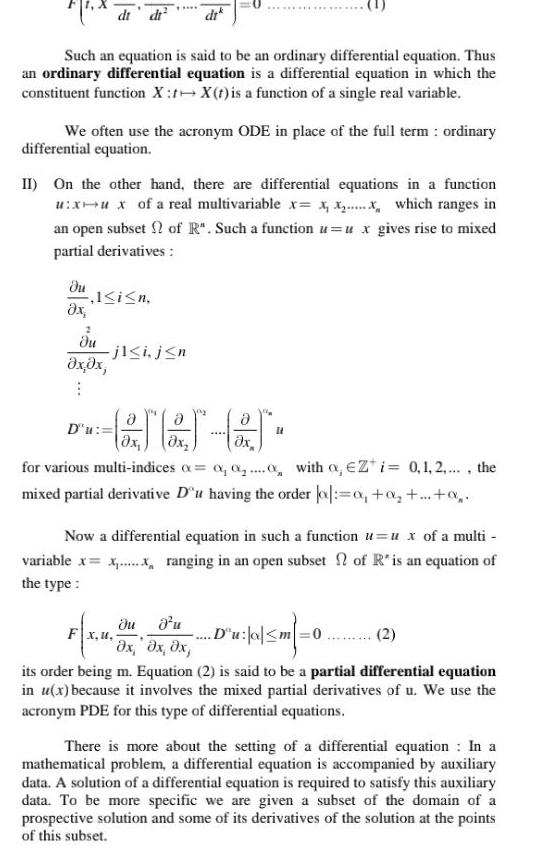Question:

# di di Such an equation is said to be an ordinary

Last updated: 9/18/2023di di Such an equation is said to be an ordinary differential equation Thus an ordinary differential equation is a differential equation in which the constituent function X X t is a function of a single real variable We often use the acronym ODE in place of the full term ordinary differential equation II On the other hand there are differential equations in a function u xu x of a real multivariable x x x x which ranges in an open subset of R Such a function u u x gives rise to mixed partial derivatives du x 1 i n Du x x jl i j n Grer er D u for various multi indices 0 0 o with o EZ i 0 1 2 the mixed partial derivative D u having the order 0 9 a Now a differential equation in such a function u ux of a multi variable x x x ranging in an open subset of R is an equation of the type du Fx u 11 d u D u ox m 0 2 its order being m Equation 2 is said to be a partial differential equation in u x because it involves the mixed partial derivatives of u We use the acronym PDE for this type of differential equations There is more about the setting of a differential equation In a mathematical problem a differential equation is accompanied by auxiliary data A solution of a differential equation is required to satisfy this auxiliary data To be more specific we are given a subset of the domain of a prospective solution and some of its derivatives of the solution at the points of this subset